U.S. Department of Transportation
1200 New Jersey Avenue, SE
Washington, DC 20590
202-366-4000

Federal Highway Administration Research and Technology
Coordinating, Developing, and Delivering Highway Transportation InnovationsThis report is an archived publication and may contain dated technical, contact, and link information
 Federal Highway Administration > Publications > Research Publications > 05081 > Design of Continuously Reinforced Concrete Pavements Using Glass Fiber Reinforced Polymer Rebars
 Publication Number: FHWA-HRT-05-081 Date: October 2005

# Chapter 5. Fe Studyof Design Consideration for Crcp

### FE Analysis of a 1.524-m (5-ft) CRCP Segment

As can be seen in figures 22 through 26, the tensile stress levels in concrete caused by both concrete shrinkage and temperature variation are far below the tensile strength of normal concrete for the free slab. Other restraining forces acting on a given concrete slab, such as friction from the subbase under concrete pavements or restraints from reinforcement ties to neighboring slabs, need to be considered. When these restraints are considered, the overall resulting tensile stress level in the concrete will increase, possibly exceeding the concrete tensile strength and causing cracks in the concrete slab.

The CRCPs considered in the FE analyses have been assumed to have transverse cracks equally spaced at 1.524 m (5 ft (60 inches)) and were subjected to the concrete shrinkage and temperature variation. Since the cracks in the CRCP are assumed to be spaced equally, the longitudinal reinforcement at the crack is constrained in both longitudinal and rotational directions. Therefore, only a representative slab segment was needed to model the CRCP. The length of the slab segment, L, is equal to the crack spacing. While longitudinal reinforcement of number 6 rebars with a radius (r) of 9.53 mm (0.375 inch) was considered in the FEM analysis, the influence of transverse reinforcement was ignored. The bond-slip behaviors between the concrete and reinforcement and between the concrete slab and subbase were modeled by using horizontal spring elements. The underlying layers are modeled as an elastic foundation with an elastic spring constant of 108.583 megapascals per meter (MPa/m) (400 poundsforce per square inch per inch (lbf/in2/inch)). The FE model of the CRCP slab segment has been assumed to be subjected to a concrete shrinkage at 28 d of 355.6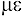and a temperature change (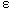T) of -27.8 °C (-50 °F) and -16.7 °C (-30 °F) at the top and bottom of the slab segment, respectively. The temperature varies linearly from the top to the bottom. All of the other model parameters and material properties used are listed in table 4, and the schematic details of the FE model are depicted in figure 44; the properties of GFRP rebar are assumed in this analysis.

Table 4. Model parameters and material properties used in FE study.
Parameter and Properties Value
Width, B (cm (inches)) 15.24 (6)
Height, H (cm (inches)) 5.40 (10)
Young's modulus of concrete at 28 d, Ec,28 (GPa (x 106 lbf/in2)) 33.10 (4.80)1 and 34.48 (5.00)2
Poisson's ratio of concrete, nc 0.20
Young's modulus of steel rebar (GPa (x 106 lbf/in2)) 200.00 (29.00)
Longitudinal Young's modulus of GFRP rebar (GPa (x 106 lbf/in2)) 40.80 (5.92)
CTE of concrete,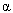c (/°C (/°F)) 10.26 (5.7)1 and 14.40 (8.0)2
CTE of steel rebar,r,s (/°C (/°F)) 11.88 (6.6)
CTE of GFRP rebar,r,g (/°C (/°F)) 9.07 (5.04)

1Granite coarse aggregate used.

2Siliceous river gravel coarse aggregate used.

1 megapoundforce per square inch (106 lbf/in2) = 6.89 gigapascal (GPa)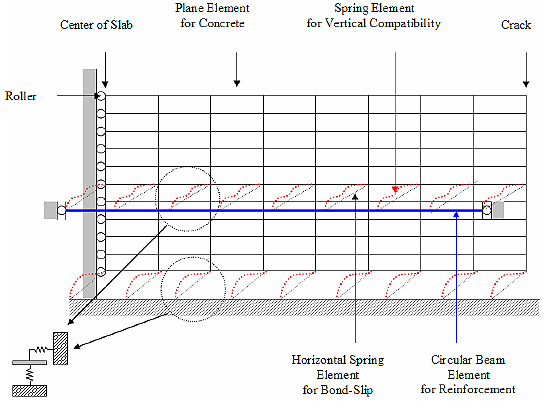Figure 44. Drawing. Schematic details of a 2-D CRCP finite element model.

In the FE analysis, the CRCP design parameters—such as the CTE of concrete, the friction from the pavement's subbase, and the bond-slip between concrete and reinforcement—were studied to assist in understanding their effects on stress development and crack width in the CRCP.

#### Effect of Concrete CTE on Stress Development in CRCP

Two different types of concrete, with granite aggregate or siliceous river gravel aggregate, have been adopted in the stress analysis of the CRCP slab segment. The CTEs of granite and siliceous river gravel concrete are 10.26/°C (5.7/°F) and 14.40/°C (8.0/°F), respectively.(30) In addition, the steel-reinforced CRCP slab segment is analyzed for comparison with the GFRP-reinforced one. The bond between the concrete and reinforcement is assumed to be perfect, and the bond-slip between the concrete slab and subbase was represented using a spring stiffness of 236.409 kilonewton per meter (kN/m) (1,350 poundsforce per inch (lbf/inch)), which is simulated for a flexible subbase or a lime-treated clay subbase, having the bond-slip stiffness per unit area of 40.719 MPa/m (150 lbf/in2/inch) with respect to the concrete slab. The spring stiffness corresponds to a concrete element with a horizontal length of 3.81 cm (1.5 inches) and a thickness in the z-direction of 15.24 cm (6 inches).

Figures 45 and 46 show the FE results of axial tensile stresses developed on the concrete surface along the longitudinal (x-) direction, considering the aforementioned restraining conditions. The maximum tensile stress level for the GFRP-reinforced slab segment at the x-midpoint, shown in figure 42, is much higher compared with those for the freely supported reinforced concrete slab, shown in figures 22 through 26. As expected, considering those restraints in the CRCP model increases the tensile stress level in concrete. As can be seen in both figures 45 and 46, because of the low Young's modulus in the GFRP rebar, the tensile stresses for the GFRP-reinforced concrete are always smaller than those for the steel ones. The use of GFRP rebar as the reinforcement might lead to larger crack spacings followed by wider crack widths in the CRCP. If it is necessary to shorten the crack spacing or narrow the crack width, it can be achieved by using a larger amount of reinforcement or by adjusting the concrete properties, the subbase restraints, or bonding between the concrete and reinforcement. For reference, AASHTO guidelines indicate that the crack spacing should be no more than 2.438 m (8 ft) to minimize the incidence of crack spalling and no less than 1.067 m (3.5 ft) to minimize the potential for the development of punchouts, and the allowable crack width should not exceed 1 mm (0.04 inch) for the steel-reinforced CRCP.(2)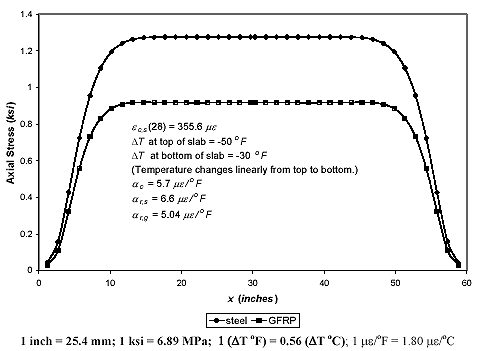Figure 45. Graph. Axial tensile stress on concrete surface versus longitudinal location of a 1.524-m (5-ft) CRCP segment with ρ = 0.00739 andc = 10.26/°C (5.7/°F).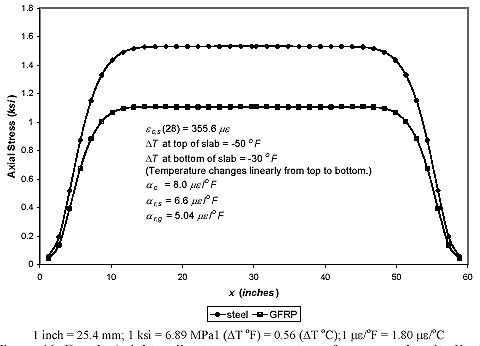Figure 46. Graph. Axial tensile stress on concrete surface versus longitudinal location of a 1.524-m (5-ft) CRCP segment with ρ = 0.00739 andc = 14.40/°C (8.0/°F).

It can be seen in the comparison between figures 45 and 46 that the tensile stress level in concrete for the granite concrete with a CTE of 10.26/°C (5.7/°F) is lower than that for the siliceous river gravel concrete with a CTE of 14.40/°C (8.0/°F). This is because the difference between CTEs of concrete and reinforcement for the granite concrete is smaller than that for the siliceous river gravel concrete. Therefore, the tensile stress in concrete can be reduced by using concrete with lower CTE. Regardless of the concrete CTEs, the maximum tensile stresses in concrete for both steel- and GFRP-reinforced slab segments are much higher than the concrete tensile strength, and all the slab segments will presumably be cracked at their midspan due to the given temperature variation and shrinkage conditions. The concrete tensile strengths for the granite and siliceous river gravel concretes are about 2.90 MPa (420 lbf/in2) and 2.55 MPa (370 lbf/in2), respectively, with a concrete compressive strength, fc′, of about 27.58 MPa (4,000 lbf/in2).

The axial stress distributions in the reinforcements along the longitudinal (x-) direction for two different concrete CTEs are shown in figures 47 and 48. While the reinforcements around the middle are under low compression, those around the crack (the ends of the slab segment) are under high tension. Because of the stiffness compatibility between GFRP and concrete, the tensile stresses in the GFRP reinforcements are lower than those in the steel reinforcements. The tensile reinforcement stress for the lower concrete CTE (figure 49) is also lower than that for the higher concrete CTE (figure 48).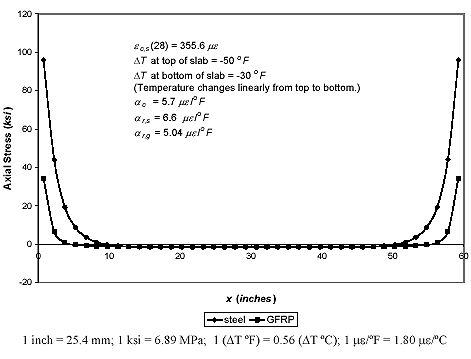Figure 47. Graph. Axial stress in reinforcement versus longitudinal location of a 1.524-m (5-ft) CRCP segment with ρ = 0.00739 andc = 10.26/°C (5.7/°F).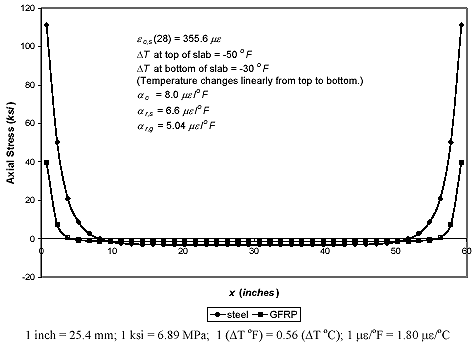Figure 48. Graph. Axial stress in reinforcement versus longitudinal location of a 1.524-m (5-ft) CRCP segment with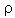= 0.00739 andc = 14.40/°C (8.0/°F).

Figures 47 and 48 show that the reinforcements are subjected to higher tensile stresses than the limits of their allowable tensile stresses. While the stress limit for the grade 60 number 6 steel rebar is about 75 percent of its ultimate tensile strength (379 MPa (55 ksi)), the GFRP rebar's allowable tensile stress is about 20 percent of its ultimate tensile strength under a harsh environment (124 MPa (18 ksi)).(2,26) The GFRP rebar's allowable stress range is established for the prevention of any undesired early failure of GFRP rebars under sustained loads due to alkaline attacks on the glass fibers.

#### Effect of Bond-Slip between Concrete Slab and Subbase

Granite aggregate concrete was adopted for this analysis. The bond between the concrete and reinforcement was again assumed to be perfect, and two different values of the bond-slip between the concrete slab and subbase were considered to study its effect on the stress development and crack width of the 1.524-m(5-ft) GFRP-reinforced CRCP slab segment; the spring stiffnesses used for the slab subbase bonds were 0.236 MN/m (1,350 lbf/inch) and 24.271 MN/m (138,600 lbf/inch). The bond-slip stiffnesses were calculated for the concrete element with a horizontal length of 3.81 cm (1.5 inches) and a thickness of 15.24 cm (6 inches), and simulated for the bond-slip of the concrete slab with respect to the flexible (lime-treated clay) subbase and the cement-stabilized subbase, respectively. While the former was calculated from the bond-slip stiffness per unit area of 40.719 MPa/m (150 lbf/in2/inch), the latter was calculated from that of 4.180 GPa/m (15,400 lbf/in2/inch).(33)

Figures 49 and 50 show the FEM results of concrete stresses at the bottom surface and top surface of the GFRP-reinforced concrete pavement along the longitudinal (x-) direction, respectively, from varying the bond-slip stiffness between the concrete and subbase. As expected, the maximum tensile stresses in the concrete caused by the shrinkage and temperature variation have drastically increased after considering the aforementioned restraining conditions. Figure 49 shows that the tensile stress in the concrete for the higher subbase friction is larger than that for the lower one at the bottom of the CRCP slab segment. At the top of the slab segment, the reversed result of the concrete stress level has been observed (figure 50); smaller stress was found for the higher friction. The higher subbase friction creates more restraint against the concrete volume change at the bottom of the slab segment. More frictional reaction force is generated at the concrete bottom, leading to less concrete displacement with larger concrete stress at the bottom. Simultaneously, this causes less concrete displacement around the reinforcement and, consequently, less reaction force provided from the reinforcement to the concrete, leading to smaller concrete stresses around the reinforcement and at the top of the slab segment.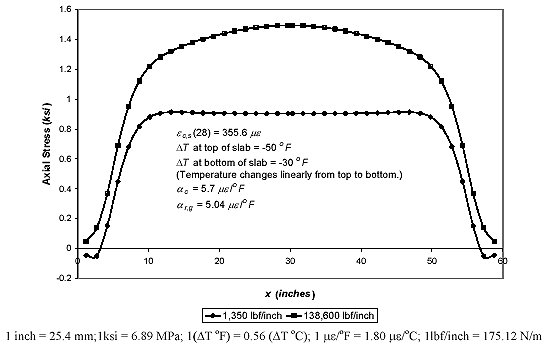Figure 49. Graph. Axial stress at concrete bottom surface versus longitudinal location for two different subbase bond stiffnesses (ρ = 0.00739 and L = 1.524 m (60 inches)).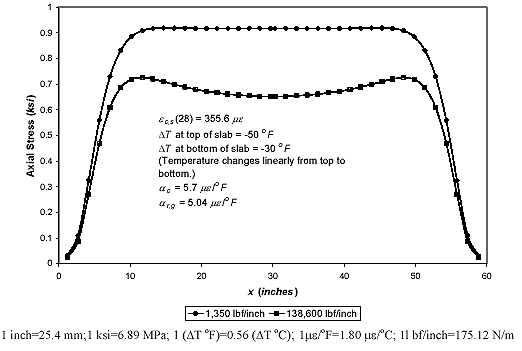Figure 50. Graph. Axial stress at concrete top surface versus longitudinal location for two different subbase bond stiffnesses (ρ = 0.00739 and L = 1.524 m (60 inches)).

Figures 51 and 52 show the axial concrete stress contours from the cases employing the two different subbase frictions. Figure 51, representing the lower friction case, shows that the stress variation is almost symmetrical with respect to the longitudinal center line on which the reinforcement is located; the stress contour is similar to that of the case without the subbase friction. On the other hand, the stress in figure 52, for the higher friction case, is observed to drastically change from the bottom to the top of the slab segment; the stress level at the bottom is much higher than that at the top. With such a high subbase friction, the CRCP is likely to have a chance to crack from its bottom area. In addition, the concrete stress at the bottom for the higher friction (figure 50) is larger than that at the top for the lower friction (figure 49). This implies that the CRCP with the higher subbase friction will have more cracks for a certain pavement length, in conjunction with smaller crack spacings. The high stress concentrations are also found around the reinforcement near the crack (the end of the slab segment) in figures 51 and 52.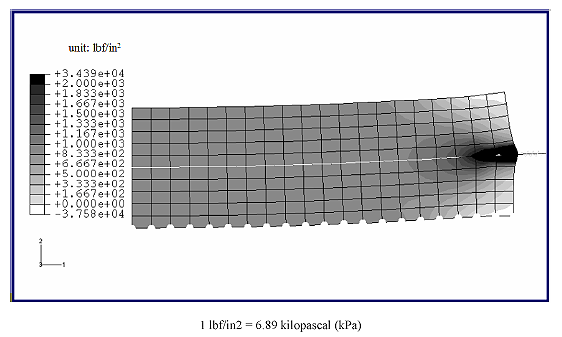Figure 51. Image. Axial stress in concrete versus longitudinal location of a 1.524-m (5-ft) CRCP segment with ρ = 0.00739, εc = 10.26/°C (5.7/°F), and a subbase bond-slip stiffness of 0.236 MN/m (1,350 lbf/inch).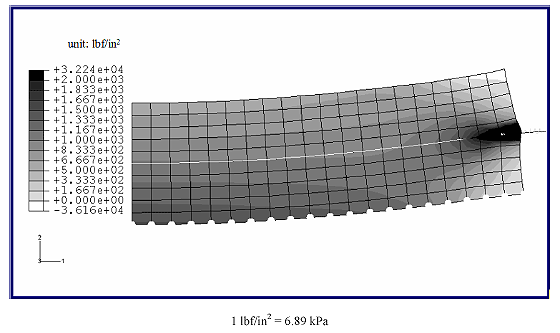Figure 52. Image. Axial stress in concrete versus longitudinal location of a 1.524-m (5-ft) CRCP segment with ρ = 0.00739, εc = 10.26/°C (5.7/°F), and a subbase bond-slip stiffness of 24.271 MN/m (138,600 lbf/inch).

The stress distributions in the GFRP reinforcement for the two different subbase frictions are plotted in figure 53. The compressive stresses (negative value in the figure) are developed around the middle of the slab segment in the longitudinal (x-) direction for both friction cases. The compressive stress level for the higher friction, about 6.46 MPa (0.94 ksi), is slightly lower than that for the lower friction, about 7.81 MPa (1.13 ksi). At the cracks, a tensile stress of about 222.31 MPa (32.24 ksi) is developed for the higher friction and is also lower than that of about 237.09 MPa (34.39 ksi) for the lower friction. As mentioned before, the subbase with the higher friction applies more frictional reaction force to the bottom of the slab segment as the concrete volume change occurs, leading to less concrete displacements both at the bottom and around the reinforcement. The lesser concrete displacement around the reinforcement releases the force applied to the reinforcement, leading to lower compressive reinforcement stresses around the middle in the longitudinal (x-) direction and lower tensile reinforcement stresses at the crack.

The variation of crack width through the depth of the slab segment is observed in figure 54. The crack width at the bottom for the higher friction is narrower than that for the lower friction because of greater restraint from the subbase. The crack width for the higher friction is narrower up to about middepth of the slab (where the reinforcement is located) and becomes wider at the top, presenting more drastic variations through the depth. In the figure, the protrusion on the graph is found at the middepth because of the restraint from the reinforcement, and the crack width at the top of the pavement is shown to be wider than that at the bottom since the magnitude of the temperature drop (T) is larger at the top than at the bottom and the restraint from the subbase exists at the bottom. The crack widths for both cases are below the allowable limitation of 1 mm (0.04 inch).(2)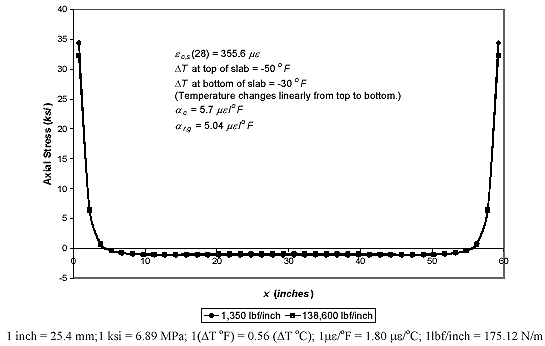Figure 53. Graph. Axial stress in GFRP rebar versus longitudinal location for two different subbase bond stiffnesses (ρ = 0.00739 and L = 1.524 m (60 inches)).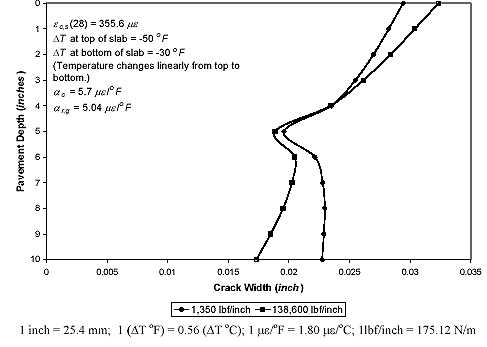Figure 54. Graph. Pavement depth versus crack width for two different subbase bond stiffnesses (ρ = 0.00739 and L = 1.524 m (60 inches)).

#### Effect of Bond-Slip between Concrete and Reinforcement

The FE analysis was also conducted for CRCP slab segments with different bond-slip stiffness between the concrete and the reinforcement. The bond-slip stiffness between concrete and reinforcement varies from 1.344 x 109 N/m (7.675 x 106 lbf/inch), 0.433 x 109 N/m (2.474 x 106 lbf/inch), and 0.175 x 109 N/m (1.0 x 106 lbf/inch), where the first value corresponds to the perfect bond case, the second corresponds to the bond-slip stiffness between the concrete and steel reinforcement, and the third is arbitrarily selected to about 40 percent of the second value. These bond-slip stiffnesses correspond to the bond-slip stiffness per unit area of 589.5 x 103 MPa/m (2.172 x 106 lbf/in2/inch), 190.0 x 103 MPa/m (0.7 x 106 lbf/in2/inch), and 76.86 x 103 MPa/m (0.283 x 106 lbf/in2/inch), respectively. A spring stiffness between the concrete slab and subbase (a flexible subbase or a lime-treated clay subbase) of 236.409 kN/m (1,350 lbf/inch), which corresponds to the bond-slip stiffness per unit area of 40.719 MPa/m (150 lbf/in2/inch), was applied for the analyses.

Figure 55 shows that the concrete stress level from a larger bond-slip stiffness is higher than that from a smaller stiffness since the tighter bond between the concrete and reinforcement provides more restraint to the concrete's volume change. It can therefore be anticipated that using reinforcement that provides the concrete with a tighter bond can cause more cracks in a certain length of CRCP, leading to smaller crack spacing and a narrower crack width. Under the given conditions, only the maximum concrete stress for the bond-slip stiffness of 0.175 x 109 N/m (1.0 x 106 lbf/inch) is below the tensile strength of the concrete. Figure 56 shows the axial stress distributions in the GFRP reinforcement for the different bond-slip stiffnesses. The stress level around the crack decreases as the bond-slip stiffness decreases. The tensile stress levels for all the bond-slip cases are higher than the allowable stress limit for the GFRP rebar. Employing reinforcement with a lower bond-slip stiffness might be one way to reduce the stress levels in both concrete and reinforcement in the CRCP. It is noted that the bond between the concrete and GFRP reinforcement cannot be perfect, and for practical purposes it can be assumed to be between the second and third bond cases. Stress development in the CRCP is sensitive to the bond between the concrete and the reinforcement.

The variation of crack width throughout the pavement depth for the crack spacing, L, of 1.524 m (5 ft) is shown in figure 57. The crack width for the higher bond-slip stiffness is narrower than that for the lower ones as a result of having more restraint against the concrete volume change. Also, the crack width for steel reinforcement is narrower than that for GFRP reinforcement at a bond-slip stiffness due to the higher Young's modulus of the steel reinforcement.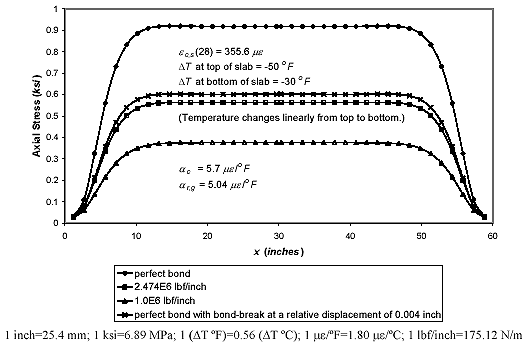Figure 55. Graph. Axial tensile stress on concrete surface versus longitudinal location for different bond-stiffnesses (ρ = 0.00739 and L = 1.524 m (60 inches)).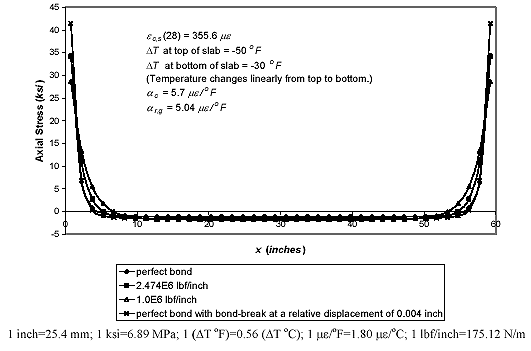Figure 56. Graph. Axial stress in GFRP rebar versus longitudinal location for different bond-stiffnesses (ρ = 0.00739 and L = 1.524 m (60 inches)).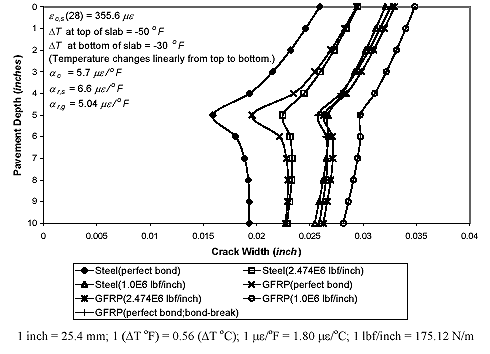Figure 57. Graph. Pavement depth versus crack width for different bond stiffnesses (ρ = 0.00739 and L = 1.524 m (60 inches)).

In figure 57, the protrusions on the graph are found at the middepth of the pavement (where the reinforcement is located), and the graph is observed to be more protruded with the higher bond-slip stiffness. This results from the fact that the concrete volume change is restrained most at the middepth by the reinforcement and is subjected to more restraint as the bond-slip stiffness increases. The crack width at the top of the pavement is wider than that at the bottom of the pavement since the magnitude of the temperature drop (T) is larger at the top than at the bottom, and the restraint from the subbase exists at the bottom. The crack widths throughout the pavement depth for all the cases are below 1 mm (0.04 inch), which is the assumed maximum allowable crack width to prevent the loss of concrete aggregate interlock.

The high stress concentration caused by the perfect bond assumption between the concrete and reinforcement, as found around the reinforcement near the crack in figures 51 and 52, is not realistic; the bond between the concrete and reinforcement will actually break near the crack, relieving the stress concentration as shown in figure 58, whose FE model is equivalent to that of figure 51 except that the bond-slip springs between concrete and reinforcement are modeled to yield when the relative spring displacement between concrete and reinforcement exceeds 0.1 mm (0.004 inch). The calculated bond-break appears at the first spring element; hence, the bond-break length is less than 3.81 cm (1.5 inches) starting from the surface of the crack. The bond-break near the crack also lowers the overall stress level in concrete (as shown in figure 58).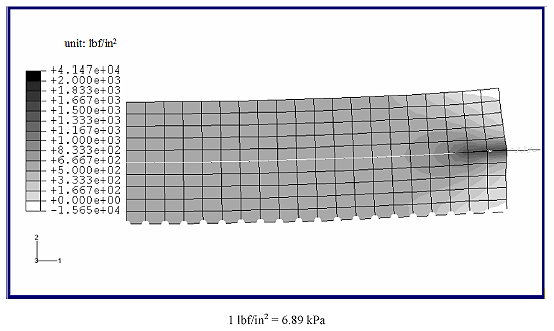Figure 58. Image. Axial stress in concrete versus longitudinal location of a 1.524-m (60-inch) CRCP segment with= 0.00739, εc = 10.26/°C (5.7/°F), and a subbase bond-slip stiffness of 236.409 kN/m (1,350 lbf/inch; bond-break considered).

This effect of bond-break can also be seen in figures 55 and 56. While the bond-break near the crack decreases the tensile concrete stress from about 6.33 MPa (0.92 ksi) to 4.15 MPa (0.60 ksi) on the concrete surface, it increases the tensile reinforcement stress from about 237.09 MPa (34.39 ksi) to 285.94 MPa (41.47 ksi) at the cracks, and the compressive reinforcement stress from about 7.81 MPa (1.13 ksi) to 10.49 MPa (1.52 ksi) at the midspan. If bond-break length increases, which will make more spring elements yield, the maximum tensile stress in the reinforcement will reduce. In addition, the effect of the bond-break on the crack width variation in the case of GFRP reinforcement is shown in figure 57. Since the bond breaks near the crack, the crack widths throughout the pavement depth become wider than those without the bond-break assumption; note that the maximum crack width of the 1.524-m (5-ft) CRCP segment is about 0.83 mm (0.033 inch) on the top surface, which is just under the allowable crack width limitation, 1 mm (0.04 inch).

### FE Analysis of CRCP Segments with Different Lengths

The previous FE results show that, in most cases, not only do the concrete stresses exceed the concrete tensile strength, but the reinforcement stresses around the crack also exceed the limit of allowable working stress of GFRP rebar under the given conditions. As previously shown, the lower bond-slip stiffness between concrete and reinforcement, as well as the smaller CTE and Young's modulus of concrete, can lower the stress levels in the concrete and reinforcement. Therefore, the bond-slip stiffness of 0.433 x 109 N/m (2.474 x 106 lbf/inch), instead of perfect bond, is employed for a design example, along with a concrete CTE of 6.84/°C (3.8/°F) and Young's modulus of 24.8 GPa (3.6 x 106 lbf/in2) for a limestone aggregate concrete. The bond-slip stiffness between concrete and subbase for flexible subbase (or lime-treated clay subbase) is applied for the example. In addition, the CRCP segments at three different lengths-1.067 m (3.5 ft), 1.524 m (5 ft), and 2.438 m (8 ft)-are modeled to represent the range of the crack spacing anticipated from the conventional CRCP design, which is 1.067 to 2.438 m (3.5 to 8.0 ft). The other conditions for the example are the same as previously adopted.

The axial tensile concrete stress distribution at the top of the slab segment, along the longitudinal direction, has been plotted for each slab length (figure 59). As shown in the figure, the concrete stress level of the longer slab segment is higher than that of the shorter slab segment. The maximum stress levels in the concrete for the lengths of 1.067 m (3.5 ft), 1.524 m (5 ft), and 2.438 m (8 ft) are about 1.86 MPa (270 lbf/in2), 3.08 MPa (447 lbf/in2), and 6.16 MPa (893 lbf/in2), respectively. The stress level for L = 2.438 m (8 ft) is well above the tensile strength, about 2.41 MPa (350 lbf/in2), of the limestone concrete approximated with a concrete compressive strength, fc′, of about 27.58 MPa (4,000 lbf/in2). This slab segment will crack at the middle. On the other hand, the slab segments with lengths shorter than 1.067 m (3.5 ft) will not crack at the middle; the maximum stress level for L = 1.067 m (3.5 ft) does not reach the tensile strength of concrete. The stress level for L = 1.524 m (5 ft) is assumed to be roughly on the verge of the cracking point or has slightly passed the point. Therefore, the crack spacing for the example is anticipated to be between 1.067 m (3.5 ft) and 1.524 m (5 ft).

Figure 60 shows the axial stress distributions in the GFRP reinforcement for the different slab segment lengths. Around the crack, the stress levels in the GFRP reach around 113.83 MPa (16.51 ksi), 192.70 MPa (27.95 ksi), and 387.59 MPa (56.21 ksi) for the lengths of 1.067 m (3.5 ft), 1.524 m (5 ft), and 2.438 m (8 ft), respectively. Except for the case of L = 1.067 m (3.5 ft), the GFRP reinforcement is subjected to higher stress levels than its limit of allowable tensile stress (124 MPa; 18 ksi); with the anticipated crack spacing of this example, shorter than 1.524 m (5 ft), the stress level could meet its limit. The variation of crack width along the pavement depth is shown in figure 61. The crack width for the longer slab segment is wider than that for the shorter one. Except for the case of L = 2.438 m (8 ft), the crack widths for all cases are below the limit of allowable crack width, 1 mm (0.04 inch).

It can be understood in this chapter that the behavior of the CRCP can be adjusted by varying the concrete material properties, subbase type, and the bond characteristics between concrete and reinforcement. For example, if it is necessary to have a smaller crack spacing in the CRCP (which can be attained by creating higher concrete stress level), concrete with a larger CTE and Young's modulus, a subbase providing the concrete slab with higher friction, reinforcement which bonds better with concrete, or a combination of the three may need to be employed. This study reveals that careful consideration of these parameters can provide favorable control of the stresses in the concrete and reinforcement followed by the control of cracking in the CRCP, allowing for a sound design of the GFRP-reinforced CRCP.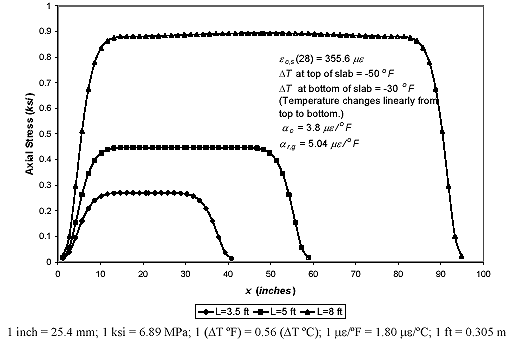Figure 59. Graph. Axial tensile stress on concrete surface versus longitudinal location for different slab segment lengths (ρ = 0.00739; bond-slip stiffness = 0.433 x 109 N/m (2.474 x 106 lbf/inch)).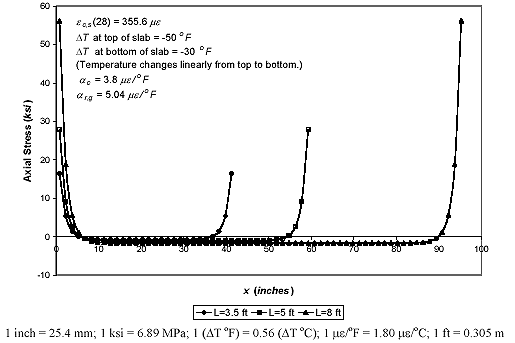Figure 60. Graph. Axial stress in GFRP rebar versus longitudinal location for different slab segment lengths (ρ = 0.00739; bond-slip stiffness = 0.433 x 109 N/m (2.474 x 106 lbf/inch)).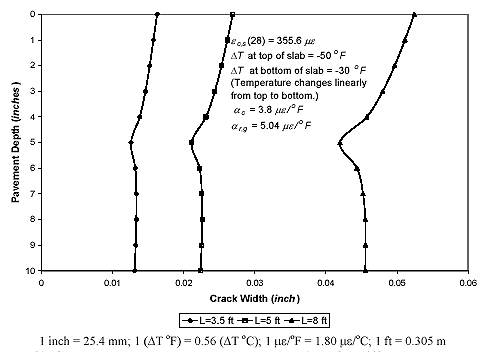Figure 61. Graph. Pavement depth versus crack width for different slab-segment lengths (ρ = 0.00739; bond-slip stiffness = 0.433 x 109 N/m (2.474 x 106 lbf/inch)).

Design considerations related to crack spacing (1.067 to 2.438 m; 3.5 to 8 ft), crack width limitation (≤1 mm (0.04 inch)), and the allowable stress limit of GFRP reinforcement were also presented in this chapter, and the results indicated that GFRP-reinforced CRCP can satisfy these requirements. For cost considerations, the reinforcement amount used in the proposed design (0.74 percent) was chosen to be the same as a traditional steel-reinforced CRCP. Typical reinforcement amounts for steel-reinforced CRCP can range between 0.5 and 0.7 percent, depending on the weather conditions.(20) Increasing the reinforcement amount will reduce the reinforcement stress, which may be a simple and necessary consideration for the GFRP-reinforced CRCP when the pavement is likely to be subjected to a harsh environment with high temperature variations and alkaline attack.

The current design example using number 6 rebars at 15.2-cm (6-inch) spacing in the longitudinal direction and number 5 at 121.9-cm (4-ft) spacing in the transverse direction in a 3.658-m- (12-ft) wide lane shows feasible performance under an extreme temperature variation (T = -27.8 °C (-50 °F) at the top surface of the pavement andT = -16.7 °C (-30 °F) at the bottom surface), with a concrete shrinkage of 355.6 me at 28 d. By careful consideration of concrete properties, proper bond between GFRP rebar and concrete, reinforcement amount, and subbase friction, the above design shows satisfactory performance.